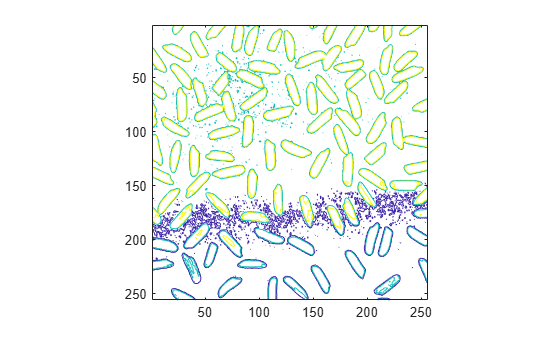## Contour Plot of Image Data

A contour is a path in an image along which the image intensity values are equal to a constant. You can create a contour plot of the data in a grayscale image using `imcontour`. This function is similar to the `contour` function in MATLAB®, but it automatically sets up the axes so their orientation and aspect ratio match the image. To label the levels of the contours, use the `clabel` function.

### Create Contour Plot of Image Data

This example shows how to create a contour plot of an image.

Read grayscale image and display it. The example uses an example image of grains of rice.

```I = imread('rice.png'); imshow(I)```Create a contour plot of the image using `imcontour` .

```figure; imcontour(I,3)```